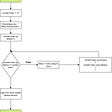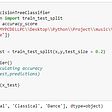# Stock Market Prediction Using Python: Article 3 ( The Prophet )

`#import packagesimport pandas as pdfrom fbprophet import Prophetimport matplotlib.pyplot as plt# function to calculate compound annual growth ratedef CAGR(first, last, periods):    return ((last/first)**(1/periods)-1) * 100# comment either of the following two blocks.# Block 1 for BSE SENSEX################################################################################# read the Indian BSE data filedf = pd.read_csv('D:\\python3\\data\\SensexHistoricalData.csv')################################################################################# Block 2 for DOW JONES################################################################################## read the US Dow Jones data file#df_i = pd.read_csv('D:\\python3\\data\\DowJonesHistoricalPrices.csv')## Dow jones data is in reverse order, i.e. from current date to the past dates.## We need to correct this before proceeding#df_i['Date'] = pd.to_datetime(df_i.Date)#df = df_i.iloc[::-1]################################################################################# preparing data. Prophet only understands y and ds columns. Hence we need to rename# our data frame columnsdf.rename(columns={'Close': 'y', 'Date': 'ds'}, inplace=True)# Model initialization. Create an object of class Prophet.model = Prophet()# Fit the data(train the model)model.fit(df)# Create a future data frame of future dates. Here 3650 is approximate number of days in 10 yrs time frame.future = model.make_future_dataframe(periods=3650)# Prediction for future dates.forecast = model.predict(future)# forecast has number of various columns. In this exercise we are considering only two of them.# ds is a date column and yhat is the median predicated value.forecast_valid = forecast[['ds','yhat']][:]forecast_valid.rename(columns={'yhat': 'y'}, inplace=True)#print the last predicted valueprint ("Closing price at 2029 would be around ", forecast_valid[['y']].iloc[-1])#print CAGR for next ten years.print ('Your investments will have a CAGR of ',(CAGR(df['y'].iloc[-1], forecast_valid[['y']].iloc[-1], 10)), '%')# create a date index for input data frame.df['Date'] = pd.to_datetime(df.ds)df.index = df['Date']# Create a date index for forecast data frame.forecast_valid['Date'] = pd.to_datetime(forecast_valid.ds)forecast_valid.index = forecast_valid['Date']# plot the actual dataplt.figure(figsize=(16,8))plt.plot(df['y'], label='Close Price History')# plot the prophet predictionsplt.plot(forecast_valid[['y']], label='Future Predictions')#set the title of the graphplt.suptitle('Stock Market Predictions "Bse Sensex"', fontsize=16)#set the title of the graph windowfig = plt.gcf()fig.canvas.set_window_title('Stock Market Predictions')#display the legendsplt.legend()#display the graphplt.show()plt.show()`

--

--

--

## More from Sumit Khedkar

Data Science and Machine Learning Expert

Love podcasts or audiobooks? Learn on the go with our new app.

## Enriching Images of Maps## Data Uniformity in Data Science## 4 Alternative Data Sources for Model Driven Investing## Monty Hall Problem Revisited## Modern Data Warehouse- Benefits and Key elements## RUBRICS CUBE? Or the secret of moving on from accidental lies and bias.## Sumit Khedkar

Data Science and Machine Learning Expert

## Mastering Python Fundamental in 3 Days | Day 3 | Looping With Python## Top 8 Most Important Unsupervised Machine Learning Algorithms With Python Code References## Python for Machine Learning: An Introduction## Starting Machine Learning with Python!